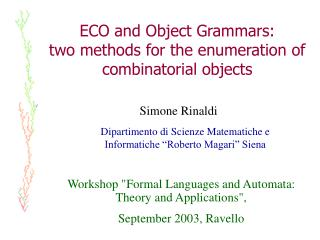Download PresentationECO and Object Grammars: two methods for the enumeration of combinatorial objects

# ECO and Object Grammars: two methods for the enumeration of combinatorial objects

Download Presentation## ECO and Object Grammars: two methods for the enumeration of combinatorial objects

- - - - - - - - - - - - - - - - - - - - - - - - - - - E N D - - - - - - - - - - - - - - - - - - - - - - - - - - -
##### Presentation Transcript

1. ECO and Object Grammars: two methods for the enumeration of combinatorial objects Simone Rinaldi Dipartimento di Scienze Matematiche e Informatiche “Roberto Magari” Siena Workshop "Formal Languages and Automata: Theory and Applications", September 2003, Ravello

2. ECO method • ECO is a method for Enumeration of Combinatorial Objects introduced by Barcucci, Del Lungo, Pergola and Pinzani O a class of combinatorial objects p a parameter on O (i.e. p: O  N+) On the objects of O having size n, On={o:p(o)=n} • ECO operator: J: OnP(On+1 ) P(On+1 ) is the set of parts of On+1

3. Proposition: Let J be an operator on O, such that: 1. for each o'On+1there iso On s.t. o'J(o); 2. for each o,o'Onwitho o', J(o) J(o') = ; then {J(o) : oOn } is a partition ofOn+1

4. A very simple example: Parallelogram Polyominoes • A parallelogram polyomino

5. A very simple example: Parallelogram Polyominoes • A parallelogram polyomino

6. A very simple example: Parallelogram Polyominoes • A parallelogram polyomino upper path lower path

7. Well-known enumerative results • The number of parallelogram polyominoes with semi-perimeter n+2: • The Catalan numbers: • 1,2,5,42,132,429,… • The 5 parallelogran polyominoes with sp=4

8. The ECO operator for Parallelogram Polyominoes (3) (1) (2) (3) (4) (k)  (1)(2) (3)…(k-1)(k)(k+1)

9. (1) (2) (1) (1) (2) (2) (3) (1) Generating tree of the operator  The recursive construction determined by  can be suitably described through a generating tree

10. fn (1) 1 (1) (2) 2 5 (1) (2) (1) (2) (3) A succession rule  (1) (k)  (1)(2)…(k)(k+1) (k+1)

11. ECO method: enumeration (1) • Born as a method for the enumeration of combinatorial objects: • E. Barcucci, A. Del Lungo, E. Pergola, R. Pinzani, ECO: a methodology for the Enumeration of Combinatorial Objects. • E. Barcucci, A. Del Lungo, E. Pergola, R. Pinzani, A methodology for plane tree enumeration. • E. Barcucci, A. Del Lungo, E. Pergola, R. Pinzani, Directed animals, forests and permutations.

12. ECO method: algebraic characterizations (2) • Operations on succession rules: • L. Ferrari, E. Pergola, R. Pinzani, S. Rinaldi, An algebraic characterization of the set of succession rules. • L. Ferrari, E. Pergola, R. Pinzani, S. Rinaldi, Jumping succession rules and their generating functions. • S. Brlek, E. Duchi, E. Pergola, S. Rinaldi, On the equivalence problem for succession rules. • Production matrices: • E. Deutsch, L. Ferrari, S. Rinaldi, Production matrices.

13. ECO method: generating functions (3) • To determine the generating function associated with an ECO operator: • C. Banderier, M. Bousquet-Melou, A. Denise, P. Flajolet, D. Gardy and D. Gouyou-Beauchamps, Generating functions for generating trees. • E. Duchi, A. Frosini, S. Rinaldi, R. Pinzani, A note on rational succession rules.

14. ECO method: random and exhaustive generation (4) • Random generation of combinatorial objects: • E. Barcucci, A. Del Lungo, E. Pergola, R. Pinzani, Random generation of trees and other combinatorial objects. • Exhaustive generation of objects: • A. Del Lungo, A. Frosini, S. Rinaldi, ECO method and the exhaustive generation of convex polyominoes. • S. Bacchelli, E. Barcucci, E. Grazzini, E. Pergola, Exhaustive generation of combinatorial objects by ECO

15. Object grammars <{Oi}iI, {EOi}iI, {j}jJ, {A}> • Oi is a class of objects; • EOi is a finite subsets of Oi (terminal objects); • j is an operation on {Oi}iI(object operation); • A is an element of {Oi}iI (axiom).

16. Object grammar for Dyck paths <{D}, {.}, {}, {D}>

17. = + Object grammar for Dyck paths <{D}, {.}, {}, {D}> f(x)=1+x2f 2(x)

18. (1) (1) (2) (1) (2) (1) (2) (3) A new approach Let L be the language of the words from the root to a node in the generating tree of  L= {(1),(1)(1), (1)(2), (1)(1)(1), (1)(1)(2), (1)(2)(1), (1)(2)(2), (1)(2)(3), … }

19. Operations on S

20. Example:

21. The series associated with  • Let C be the series associated with : C=(1)+(1)C +(1)C +(1)CC • Let wC, w (1) : w = (1)(1)(2)(3)(3)  (1)C w = (1)(2)(2)(3)(4)(2)  (1)C w = (1)(2)(2)(3)(4)(2)(1)(2)(2)  (1)C C

22. Formal power series and Object Grammars C=(1)+(1)C +(1)C +(1)CC C(x)=x+xC(x) +xC(x)+xC2

23. Formal power series and Object Grammars C=(1)+(1)C +(1)C +(1)CC

24. Formal power series and Object Grammars C=(1)+(1)C +(1)C +(1)CC (1)(1)(2)(3)(3) (1)

25. Formal power series and Object Grammars C=(1)+(1)C +(1)C +(1)CC (1)(1)(2)(3)(3) (1)(1)

26. Formal power series and Object Grammars C=(1)+(1)C +(1)C +(1)CC (1)(1)(2)(3)(3) (1)(1)(2)

27. Formal power series and Object Grammars C=(1)+(1)C +(1)C +(1)CC (1)(1)(2)(3)(3) (1)(1)(2)(3)

28. Formal power series and Object Grammars C=(1)+(1)C +(1)C +(1)CC (1)(1)(2)(3)(3) (1)(1)(2)(3)(3)

29. C Formal power series and Object Grammars C=(1)+(1)C +(1)C +(1)CC

30. C Formal power series and Object Grammars C=(1)+(1)C +(1)C +(1)CC

31. C C Formal power series and Object Grammars C=(1)+(1)C +(1)C +(1)CC

32. Results • Object grammars for various classes of polyominoes • Directed-convex polyominoes • Directed column-convex polyomines • Column-convex polyominoes • Convex polyominoes

33. Operations for column-convex polyominoes (class C)

34. Operations for the class B

35. Operations for the class P

36. Operations for the class Q